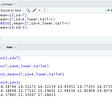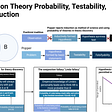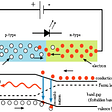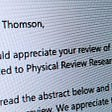# The Mathematical Universe

If you ask me what is the universe made of, I will say “Mathematics” while some people might argue that mathematics is used to describe only the physical properties of the universe but I believe that the universe itself is Math.

Everything in the universe including humans can be represented by a mathematics equation. Every shape in the universe is a part of a mathematical structure. All matter is made up of particles, which have properties such as charge and spin but these properties are purely mathematical. Space itself has properties such as dimensions but still ultimately a mathematical structure.

All things from the distance of the Earth from the Sun, to single atoms, to our DNA, can be represented by mathematical or abstract algebraic equations.

The universe does not consist of particles but is mathematical in nature which is constituted by space, time, matter and energy. All the objects in the universe are defined by virtue of certain quantifiable attributes like mass, length, volume, or position. The standard model of particle physics shows that everything that exists is composed of only three fundamental forces (electromagnetism, gravity and strong force) and four primary particles (quarks, leptons and bosons).

Mathematics is a physical phenomenon that describes anything. It’s a language that describes our universe and how it works. For scientific discovery to move from the realm of theory to fact, it must be proven through empirical inquiry. The key to that endeavor is the necessity of reproducibility, allowing for reproducible results across sample populations. Technological development has made reproducibility possible for laboratories set up with precise standards, laboratory equipment, state-of-the-art instrumentation, trained personnel, and precise protocols. Enormous strides have been made in this regard over the past decade, helping scientists discover new phenomena previously.

If you observe the universe/nature you will find out that the universe is full of patterns and everything in the universe has patterns even neurons in the human brain. With that, we come to one of the most complex structures in the universe that is “Human Brain”.

Do you know how a machine learning model works? It learns exactly like a human brain. It takes the data and finds patterns in the data. Then it processes the information and makes a mathematical equation out of it. Even though the brain is very complex it can be represented in mathematics. Even Randomness can be described and proved in mathematics.

According to me, the brain is just mathematics, If you try to simulate a computer what a person will do we just need to give enough data to the computer and it will exactly predict what the person is going to do before the event occurs.

Even consciousness is purely mathematical, Everything in the universe that takes some kind of data as input, processes the data and gives output is conscious. And yes you are right according to this definition Computers are also conscious.

The nonliving world also behaves in a mathematical way. If we throw a ball in the air it follows a parabolic trajectory. Planets and other astrophysical bodies follow elliptical orbits.

By connecting everything from the speed of light to the motion of a single atom to an algebraic equation, Universe gives you a new appreciation of the cosmos. It also proposes that mathematical laws that govern our universe can be used to describe almost anything in it.

Mathematics is the science of finding relationships between things, patterns, systems, relationships. The artform of mathematics is finding joy in these discoveries no matter how simple or complex. As the old saying goes, ‘The numbers prove the wisdom’.

--

--

--

## More from Ashish Kumar Jagrawal

Computer Scientist who is curious to know everything about the universe.

Love podcasts or audiobooks? Learn on the go with our new app.

## How Mathematical Proofs can Help Unlock the Secrets of the Brain## “Computational Complexity” Science-Research, November 2021 — summary from Arxiv and Astrophysics…## Naïve Baye’s Theorem (for the Slow) — Part 1## Quantum Wheel## Normal Distribution in R## There is a mathematical equation that proves the existence of God## Ashish Kumar Jagrawal

Computer Scientist who is curious to know everything about the universe.

## The Allegory of Applied Mathematics## Popper on Theory Probability, Testability, and Induction## INTRODUCTION## So You’ve Been Asked To Review A Paper… | Dr S J Thomson